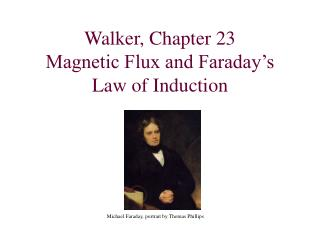Download PresentationWalker, Chapter 23 Magnetic Flux and Faraday’s Law of Induction# Walker, Chapter 23 Magnetic Flux and Faraday’s Law of Induction - PowerPoint PPT Presentation

Download Presentation##### Walker, Chapter 23 Magnetic Flux and Faraday’s Law of Induction

Download Policy: Content on the Website is provided to you AS IS for your information and personal use and may not be sold / licensed / shared on other websites without getting consent from its author. While downloading, if for some reason you are not able to download a presentation, the publisher may have deleted the file from their server.

- - - - - - - - - - - - - - - - - - - - - - - - - - - E N D - - - - - - - - - - - - - - - - - - - - - - - - - - -
##### Presentation Transcript

1. Walker, Chapter 23Magnetic Flux and Faraday’s Law of Induction Michael Faraday, portrait by Thomas Phillips

2. Induced EMF (Voltage) from changing Magnetic Flux Electric currents produce magnetic fields. 19th century puzzle, can magnetic fields produce currents? A static magnet will produce no current in a stationary coil Faraday: If the magnetic field changes, or if the magnet and coil are in relative motion, there will be an induced EMF (and therefore current) in the coil. Key Concept: The magnetic flux through the coil must change, this will induce an EMF e in the coil, which produces a current I = e/ R in the coil. Such a current is said to be induced by the varying B-field.

3. Magnetic Flux For a “loop” of wire (not necessarily circular) with area A, in an external magnetic field B,the magnetic flux is: q

4. Walker problem # 2 A uniform magnetic field of 0.0250 T points vertically upward. Find the magnitude of the magnetic flux through each of the five sides of the open-topped rectangular box shown in the figure, given that the dimensions of the box are L = 31.5 cm, W = 12.0 cm, and H = 10.0 cm.

5. Question: Magnetic Field from loop • Current I flows around the loop as shown, driven by the battery. Inside the loop the magnetic field generated by current I is: • Out of screen • Into screen

6.        Magnetic flux generated by current in loop. •   •   •   • In this case, we choose clockwise to be positive current. • In the plane of this picture, the magnetic field generated by this current is into the picture inside the loop, and out of the picture outside of the loop.

7. Examples of Induced Current Any change of current in primary induces a current in secondary. The secondary shows a deflection only during the instant just after the switch is closed or opened.

8. Induction by Relative Motion v • When a permanent magnet moves relative to a coil, the magnetic flux through the coil changes, inducing an EMF in the coil. • In a) the flux is increasing • In c) the flux is decreasing in magnitude. • In a) and c) the induced current has opposite sign. v

9. Faraday’s Law of InductionLenz’s Law Faraday’s Law: The instantaneous EMF in a circuit (w/ N loops) equals the rate of change of magnetic flux through the circuit:

10. Question: Changing Magnetic Flux • This is a plot of magnetic flux (Wb=T·m2) through a coil. • For which interval (a, b, or c) is the change in flux 0? c a b

11. Walker, Problem 23-9 • A 0.25 T magnetic field is perpendicular to a circular loop of wire with 50 turns and a radius 15 cm. • The magnetic field is reduced to zero in 0.12 s. • What is the magnitude of the induced EMF? (answer 7.35 V)

12. Inductance • Consider a solenoid of length l with N windings and radius r (Area A=p r2). • A current I produces a magnetic field in the solenoid of B = m0 N I / l • This produces a total flux through each winding of F = A B = (m0 N A / l ) I • Define the Inductance L = (m0 N2 A / l ) (not a length!!) • If the current in the solenoid changes, there will be an induced EMF • e = - N DF/Dt = - (m0 N2 A / l ) (DI/Dt) • e = - L (DI/Dt)

13. Inductance Walker Problem #42 Determine the inductance of a solenoid with 650 turns in a length of 25 cm. The circular cross section of the solenoid has a radius of 4.0 cm. [answer: 10.7 mH]

14. Example of Inductance • Consider Solenoid from previous example, • Radius r = 5cm, length l = 10 cm, 3500 windings. • L = (m0 N2 Area / l ) = (m0 N2p r2 / l )

15. Transformers • Microscopic currents in soft iron yoke amplify magnetic field produced by coils, and contain all magnetic field lines to “magnetic circuit” formed by iron. • Both primary and secondary circuits experience the same magnetic flux for each winding, but have different number of windings.

16. Flux in Transformer F = flux through a single winding or either coil. (produced by currents in either or both circuits) EMF(primary) = - NPDF/Dt EMF(secondary) = - NSDF/Dt eP / eS = NP / NS Energy conservation requires eP IP = eS IS IP / IS = NS / NP

17. Walker problem #61 A step-up transformer has 20 turns on the primary coil and 500 turns on the secondary coil. If this transformer is to produce an output of 4600 V with a 12 mA current, what input current and voltage are needed? [answer: 0.3 A, 184 V]